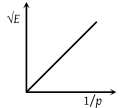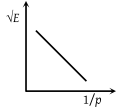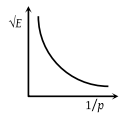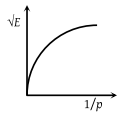The graph between $\sqrt{E}$ and $\frac{1}{p}$ is (E = kinetic energy and p = momentum)

(1)(2)(3)(4)Concept Videos :-

#1 | Work: Definition
#2 | Solved Problems

Concept Questions :-

Concept of work

(3) $P=\sqrt{2mE}$ it is clear that $P\propto \sqrt{E}$

So the graph between P and $\sqrt{E}$ will be straight line.

but graph between $\frac{1}{P}$ and $\sqrt{E}$ will be hyperbola

Difficulty Level:

• 14%
• 17%
• 58%
• 14%